Asymptotic pseudounitary stacking operatorsNext: LEAST-SQUARES INVERSION AND ADJOINT Up: Asymptotic pseudounitary stacking operators Previous: THEORETICAL DEFINITION OF A

# ASYMPTOTIC INVERSION: RECONSTRUCTING THE DISCONTINUITIES

Mathematical analysis of the inverse problem for operator (1) shows that only in rare cases can we obtain an analytically exact inversion. A well-known example is the Radon transform, which has acquired a lot of different aliases in geophysical literature: slant stack, tau-p transform, plane wave decomposition, and controlled directional reception (CDR) transform (Gardner and Lu, 1991). In this case,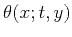(2)(3)

Radon obtained a result similar to the theoretical inversion of operator (1) with the summation path (2) and the weighting function (3) in 1917, but his result was not widely known until the development of computer tomography. According to Radon (1917), the inverse operator has the form(4)

where(5)(6)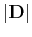is a one-dimensional convolution operator with the spectrum:(7)

andis the dimensionality of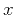and(usually 1 or 2). In Russian geophysical literature, a similar result for the inversion of the CDR transform was published by Nakhamkin (1969).

Extension of Radon's result to the general form of integral operator (1) (generalized Radon transform) is possible via asymptotic analysis of the inverse problem. In the general case, Beylkin (1985) and Goldin (1988) have shown that asymptotic inversion can reconstruct discontinuous parts of the model. These are the parts responsible for the asymptotic behavior of the model at high frequencies. Since the discontinuities are associated with wavefronts and reflection events at seismic sections, there is a certain correspondence between asymptotic inversion and such standard goals of seismic data processing as kinematic equivalence and amplitude preservation.

The main theorem of asymptotic inversion can be formulated as follows (Goldin, 1988). The leading-order discontinuities inare reconstructed by an integral operator of the form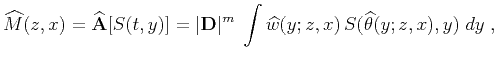(8)

where the summation pathis obtained simply by solving the equation(9)

for(if such an explicit solution is possible). The correctly chosen summation path reconstructs the geometry of the discontinuities. To recover the amplitude, we must choose the correct weighting function, which is constrained by the equation (Goldin, 1988; Beylkin, 1985)(10)

where(11)(12)

The solution assumes that differential formsandexist and are bounded and non-vanishing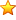. In the multi-dimensional case, they are replaced by the determinants of the corresponding matrices. To ensure the asymptotic inversion, equation (10) must be satisfied at least in the vicinity of the stationary points of integral (1). Those are the points where the summation path of the form (9) is tangent to the traveltimes of the actual events on the transformed model. In the case of the Radon transform,, and the asymptotic inverse coincides with the exact inversion.Asymptotic pseudounitary stacking operatorsNext: LEAST-SQUARES INVERSION AND ADJOINT Up: Asymptotic pseudounitary stacking operators Previous: THEORETICAL DEFINITION OF A

2013-03-03